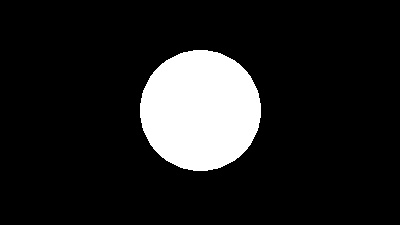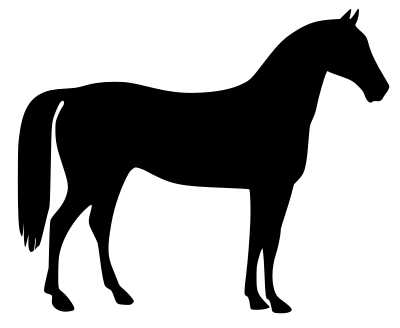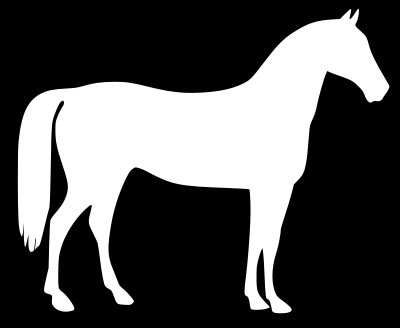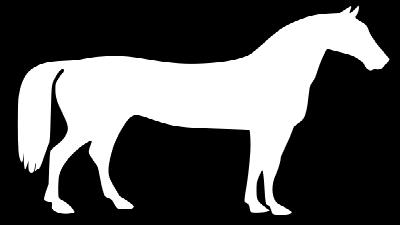# Create transparent png image with Python, Pillow (putalpha)

Posted: 2019-05-14 / Tags: Python, Pillow, Image Processing

`Image` module of the Python image processing library Pillow (PIL) provides `putalpha()` for adding an alpha channel to an image.

Here, the following contents will be described.

• How to use `Image.putalpha()`
• Set uniform transparency over the entire surface
• Create alpha channel in drawing
• Use existing image as alpha channel

Please refer to the following article for the installation and basic usage of Pillow (PIL).

Import `Image` from `PIL` and load the original image.

`ImageDraw` and `ImageFilter` are used to draw shapes and create alpha channels. They may be omitted if the image file is read and used as an alpha channel.

``````from PIL import Image, ImageDraw, ImageFilter

im_rgb = Image.open('data/src/lena.jpg')
``````## How to use Image.putalpha()

The parameter of `putalpha()` is only `alpha`. As it is literally put the alpha channel layer to the original image.

If `mode` of the original image is `RGB` (8 bit x 3: full color) or `L` (8 bit x 1: black and white), an alpha channel is newly added, and if `RGBA` or `LA`, the original alpha channel is updated.

### Specified by a constant

When an integer value of 8 bits (`0` to `255`) is set to `alpha`, the transmittance according to the value is set on the entire surface.

`0` means 100% transparency, `255` means 0% transparency (no transparency).

### Specified by Image object

If you set an `Image` object of the same size as the original image in `mode='L'` (8-bit grayscale) to `alpha`, you can set the alpha channel to various shapes.

As in the constant case, `0` means 100% transparency, `255` means 0% transparency (no transparency).

## Set uniform transparency over the entire surface

If you set an integer value to alpha, the transparency according to the value will be set on the entire surface.

Note that `putalpha()` overwrites the original image, so if you want to keep the original image as it is, use the one copied with `copy()`.

``````im_rgba = im_rgb.copy()
im_rgba.putalpha(128)
im_rgba.save('data/dst/pillow_putalpha_solid.png')
``````In this example, `alpha=128` gives about 50% transparency (128 / 255 ~ 50%).

## Create alpha channel in drawing

If you want to add an alpha channel with a simple shape, such as a circle or rectangle, drawing in the `ImageDraw` module is useful. For details on drawing, see the following article. You can also draw polygons.

Draw a white circle on a black background and set it to the alpha channel.

``````im_a = Image.new("L", im_rgb.size, 0)
draw = ImageDraw.Draw(im_a)
draw.ellipse((140, 50, 260, 170), fill=255)
````````````im_rgba = im_rgb.copy()
im_rgba.putalpha(im_a)
im_rgba_crop = im_rgba.crop((140, 50, 260, 170))
im_rgba_crop.save('data/dst/pillow_putalpha_circle.png')
``````You can use `ImageFilter` to smooth out the boundaries.

``````im_a_blur = im_a.filter(ImageFilter.GaussianBlur(4))
````````````im_rgba = im_rgb.copy()
im_rgba.putalpha(im_a_blur)
im_rgba_crop = im_rgba.crop((135, 45, 265, 175))
im_rgba_crop.save('data/dst/pillow_putalpha_circle_blur.png')
``````In each example, the outside of the circle is trimmed to a rectangle with `crop()`. See the following article for `crop()`.

## Use existing image as alpha channel

You can load an existing image and set it as an alpha channel.

Try using a black and white horse-shaped image (scikit-image sample: skimage.data.horse()).In this example, to leave the image horse-shaped, use an image with a white horse and a black background (a inverted image).After the image is read by `open()`, it is adjusted to the size of the pasted image by `resize()`, and the mode is converted to `'L'` (gray scale) by `convert()`.

``````im_a = Image.open('data/src/horse_r.png').convert('L').resize(im_rgb.size)
````````````im_rgba = im_rgb.copy()
im_rgba.putalpha(im_a)
im_rgba.save('data/dst/pillow_putalpha_horse.png')
``````